# Time and Work

#### Video Lesson on Time and Work

Work is always considered as an entire value or one. There exists an analogy between the time-speed-distance problems and work. Work based problems are more or less related to time speed and distance.

### Important Formulae

1) Work from days:
• 1) If a person can do a work in 'n' days, then person's 1 day work = 1 / n
• 2) Days from work: If a person's 1 day work is equal to 1/n , then the person can finish the work in 'n' days.
• 3)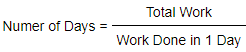### Quick Tricks and Tips:

#### 1) Ratio:

If 'A' is 'x' times as good a workman as 'B', then
a) Ratio of work done by A & B in equal time = x: 1
b) Ratio of time taken by A & B to complete the work = 1: x. This means that 'A' takes (1/xth) time as that of 'B' to finish same amount of work.
For example
if A is twice good a workman as B, then it means that
a) A does twice as much work as done by B in equal time i.e. A:B = 2:1
b) A finishes his work in half the time as B#### 2) Combined Work

a) If 'A' and 'B' can finish the work in 'x' & 'y' days respectively, then
A's one day work = 1/x
B's one day work = 1/y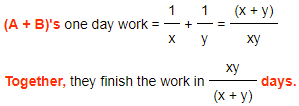b)If 'A', 'B' & 'C' can complete the work in x, y & z days respectively, then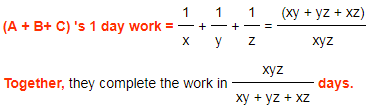c) If A can do a work in 'x' days and if the same amount of work is done by A & B together in 'y' days, then
A's one day work = 1/x
(A+B)'s one day work = 1/y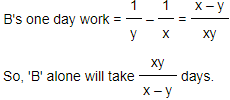d) If A & B together perform some part of work in 'x' days, B & C together perform it in 'y' days and C & A together perform it in 'z' days, then
(A + B)'s one day work = 1/x
(B + C)'s one day work = 1/y
(C + A)'s one day work = 1/z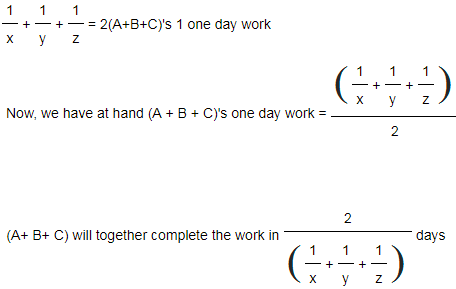If A works alone, then deduct A's work from the total work of B & C to find the time taken by A alone.
For A working alone, time required =A's work - (A+B+C)'s combined work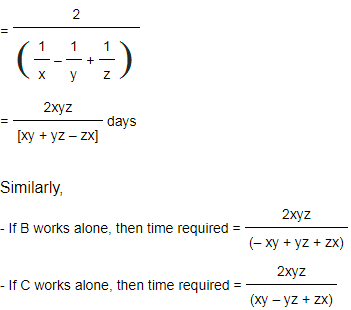#### 3) Man -Work -Hour related problems

Remember that M D H/ W= Constant
where,
M: Number of Men
D: Number of Days
H: Number of Hours
W: Amount of Work done

If men are fixed, work is proportional to time. If work is fixed , time is inversely proportional to men. Thus,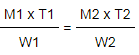Once you have understood the following simple things, this chapter will become extremely easy for you.
a) Work and time are directly proportional to each other
b) Number of men and time are inversely proportional to each other
c) And, work can be divided into equal parts i.e. if a task is finished in 10 days, in one day you will finish (1/10th) part of the work.

### About us

EntryTest.com is a free service for students seeking successful career.CAT - College of Admission Tests. All rights reserved. College of Admission Tests Online Test Preparation The CAT Online